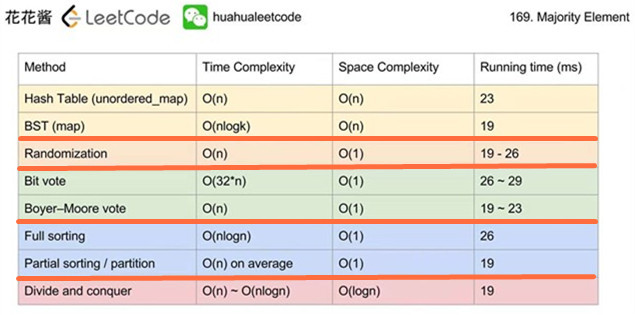# 169_求众数（Majority-Element）## 描述

输入: [3,2,3]



输入: [2,2,1,1,1,2,2]



### Java 实现

class Solution {
public int majorityElement(int[] nums) {
int majorityCount = nums.length / 2;
for (int num1 : nums) {
int count = 0;
for (int num2 : nums) {
if (num2 == num1) {
++count;
}
}
if (count > majorityCount) {
return num1;
}
}
throw new IllegalArgumentException("The array does not contain a majority element!");
}
}


### Python 实现

class Solution:
def majorityElement(self, nums):
"""
:type nums: List[int]
:rtype: int
"""
majority_count = len(nums) // 2
for num1 in nums:
count = sum(1 for num2 in nums if num2 == num1)
if count > majority_count:
return num1


### 复杂度分析

• 时间复杂度：$O(n^2)$，其中 $n$ 表示数组的长度，由于嵌套了两层 for 循环，因此总的时间复杂度是 $O(n^2)$
• 空间复杂度：$O(1)$

## 解法二：哈希表

### Java 实现

class Solution {
public int majorityElement(int[] nums) {
Map<Integer, Integer> counts = new HashMap<>();
for (int num : nums) {
if (counts.containsKey(num)) {
counts.replace(num, counts.get(num) + 1);
} else {
counts.put(num, 1);
}
}

Map.Entry<Integer, Integer> majorityEntry = null;
for (Map.Entry<Integer, Integer> entry : counts.entrySet()) {
if (majorityEntry == null || entry.getValue() > majorityEntry.getValue()) {
majorityEntry = entry;
}
}

return majorityEntry.getKey();
}
}


### Python 实现

class Solution:
def majorityElement(self, nums):
"""
:type nums: List[int]
:rtype: int
"""
counts = dict()
for num in nums:
counts[num] = counts.get(num, 0) + 1
return max(counts, key=counts.get)


### 复杂度分析

• 时间复杂度：$O(n)$，其中 $n$ 为数组的长度。由于哈希表中元素的数目最多为 $n - \left( \left \lfloor n/2 \right \rfloor + 1 \right) + 1 = n - \left \lfloor n/2 \right \rfloor$，因此遍历一次哈希表最多需要 $n - \left \lfloor n/2 \right \rfloor$ 次操作，而遍历一遍数组需要 $n$ 次操作，所以总的时间复杂度是 $O(n)$
• 空间复杂度：$O(n)$，因为哈希表最多需要保存 $n - \left \lfloor n/2 \right \rfloor$ 个元素

## 解法三：排序

### Java 实现

class Solution {
public int majorityElement(int[] nums) {
Arrays.sort(nums);
return nums[nums.length / 2];
}
}


### Python 实现

class Solution:
def majorityElement(self, nums):
"""
:type nums: List[int]
:rtype: int
"""
return sorted(nums)[len(nums) // 2]


### 复杂度分析

• 时间复杂度：$O(n \log(n))$，其中 $n$ 表示数组的长度，对数组进行排序的时间复杂度为 $O(n \log(n))$
• 空间复杂度：$O(n)$ 或者 $O(1)$，取决于是否可以直接对原数组直接进行排序，如果不允许的话，需要额外的空间复制数组

## 解法六：多数投票算法（Boyer-Moore majority vote algorithm）

### Java 实现

class Solution {
public int majorityElement(int[] nums) {
int me = nums, count = 1;
for (int i = 1; i < nums.length; ++i) {
if (count == 0) {
me = nums[i];
count = 1;
} else if (me == nums[i]) {
++count;
} else {
--count;
}
}
return me;
}
}


### Python 实现

class Solution:
def majorityElement(self, nums):
"""
:type nums: List[int]
:rtype: int
"""
me, count = 0, 0
for num in nums:
if count == 0:
me, count = num, 1
elif me == num:
count += 1
else:
count -= 1
return me


### 复杂度分析

• 时间复杂度：$O(n)$，其中 $n$ 表示数组的长度
• 空间复杂度：$O(1)$
posted @ 2018-10-31 10:00  xugenpeng  阅读(826)  评论(0编辑  收藏